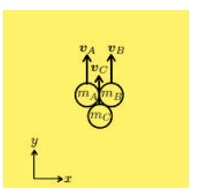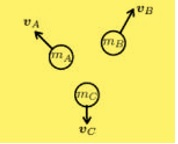A fireworks display, is made from three masses ( m A = m B = 100 g , m C = 200 g) as shown....

Question:

A fireworks display, is made from three masses ({eq}m_A = m_B = 100g, m_C = 200 {/eq}g) as shown. Gravity points down.(a) The fireworks display is held together and launched vertically upwards from the ground with initial velocity {eq}\upsilon_A = \upsilon_B = \upsilon_C = 100 {/eq}{eq}ms^-1 {/eq}. Determine the maximum height relative to ground of the three masses if joined for the whole duration.

(b) Now, the fireworks display is launched as described in part (a), but the masses then split. Just before the split, the velocity is given by {eq}\upsilon_A = \upsilon_B = \upsilon_C = 2ms^-1 {/eq} j. Just after the split, the velocity of A is given by {eq}\upsilon_A = -5ms^-1 i + 5ms^-1 {/eq} j and the velocity of C is given by {eq}\upsilon_C = -2ms^-1 {/eq} j. Determine the velocity of B just after the split.(c) Determine the maximum height relative to ground of the centre of mass after the split described in part (b).

(d) Determine the total energy required for the split described in part (b) to occur.

Energy Conservation:

Energy Conservation principle state that the energy of the system remains constant through out the process. It means that the energy of the system at initially is equals to the energy of the system finally.

Given Data

• The mass of A is: {eq}{m_A} = 100\;{\rm{g}} {/eq}.
• The mass of B is: {eq}{m_B} = 100\;{\rm{g}} {/eq}.
• The mass of C is: {eq}{m_C} = 200\;{\rm{g}} {/eq}.
• The velocity of the all three masses is: {eq}{v_A} = {v_B} = {v_C} = 100\;{\rm{m/s}} {/eq}.

(a)

The expression for the maximum height relative to the ground is,

{eq}h = \dfrac{{{v^2}}}{{2g}} {/eq}

Substitute the given values.

{eq}\begin{align*} v &= \dfrac{{{{100}^2}}}{{(2 \times 9.81)}}\\ &= 509.68\;{\rm{m}} \end{align*} {/eq}

Thus the maximum height relative to the ground is {eq}509.68\;{\rm{m}} {/eq}.

(b)

The expression for the momentum conservation is,

{eq}{m_a}\;{u_a}\; + {\rm{ }}{m_b}\;{u_b}\; + {\rm{ }}{m_c}\;{u_c}\;\; = {\rm{ }}{m_a}\;{v_a}\; + {\rm{ }}{m_b}\;{v_b}\; + {\rm{ }}{m_c}\;{v_c} {/eq}

Substitute the given values.

{eq}\begin{align*} 0.1{\rm{ }} \times {\rm{ }}2{\rm{ }}j{\rm{ }} + {\rm{ }}0.1{\rm{ }} \times {\rm{ }}2{\rm{ }}j{\rm{ }} + {\rm{ }}0.2{\rm{ }} \times {\rm{ }}2{\rm{ }}j{\rm{ }} &= {\rm{ }}0.1{\rm{ }} \times {\rm{ }}\left( {{\rm{ }} - {\rm{ }}5{\rm{ }}i{\rm{ }} + {\rm{ }}5{\rm{ }}j{\rm{ }}} \right){\rm{ }} + {\rm{ }}0.1{\rm{ }} \times {\rm{ }}{v_b}\;\; + {\rm{ }}0.2 \times \left( {{\rm{ }} - {\rm{ }}2{\rm{ }}j{\rm{ }}} \right)\\ 0.8{\rm{ }}j{\rm{ }} &= {\rm{ }} - {\rm{ }}0.5{\rm{ }}i{\rm{ }} + {\rm{ }}0.5{\rm{ }}j{\rm{ }} - {\rm{ }}0.4{\rm{ }}j{\rm{ }} + {\rm{ }}0.1{\rm{ }}{v_b}\\ {v_b}\; &= {\rm{ }}5{\rm{ }}i{\rm{ }} + {\rm{ }}7{\rm{ }}j \end{align*} {/eq}

Thus the velocity of B just after the split is {eq}{\rm{ }}5{\rm{ }}i{\rm{ }} + {\rm{ }}7{\rm{ }}j {/eq}.

(c)

The momentum of the system conserved thus the center of the mass does not change after the split.

(d)

The expression for the total energy required for the split described in part (b) to occur is,

{eq}E = {m_A}\left( {v_{Ax}^2 + v_{Ay}^2} \right) + {m_B}\left( {v_{Bx}^2 + v_{By}^2} \right) + {m_C}\left( {v_{Cx}^2 + v_{Cy}^2} \right) - {m_A}v_{Ay}^2 - {m_B}v_{By}^2 - {m_C}v_{Cy}^2 {/eq}

Substitute the given values.

{eq}\begin{align*} E &= \left( {100\;{\rm{g}}\left( {\dfrac{{1\;{\rm{kg}}}}{{1000\;{\rm{g}}}}} \right)} \right)\left( {{5^2} + {5^2}} \right) + \left( {100\;{\rm{g}}\left( {\dfrac{{1\;{\rm{kg}}}}{{1000\;{\rm{g}}}}} \right)} \right)\left( {{5^2} + {7^2}} \right) + \left( {100\;{\rm{g}}\left( {\dfrac{{1\;{\rm{kg}}}}{{1000\;{\rm{g}}}}} \right)} \right)\left( {{2^2}} \right) - \left( {100\;{\rm{g}}\left( {\dfrac{{1\;{\rm{kg}}}}{{1000\;{\rm{g}}}}} \right)} \right){\left( 2 \right)^2} - \left( {100\;{\rm{g}}\left( {\dfrac{{1\;{\rm{kg}}}}{{1000\;{\rm{g}}}}} \right)} \right){\left( 2 \right)^2} - \left( {200\;{\rm{g}}\left( {\dfrac{{1\;{\rm{kg}}}}{{1000\;{\rm{g}}}}} \right)} \right){\left( 2 \right)^2}\\ &= 11.6\;{\rm{J}} \end{align*} {/eq}

Thus the total energy required for the split is {eq}11.6\;{\rm{J}} {/eq}.